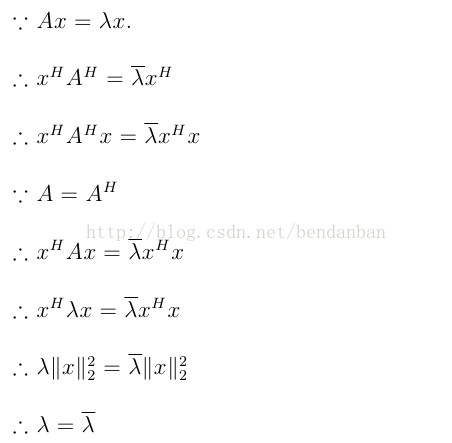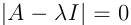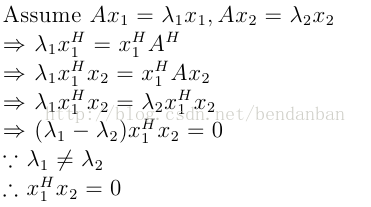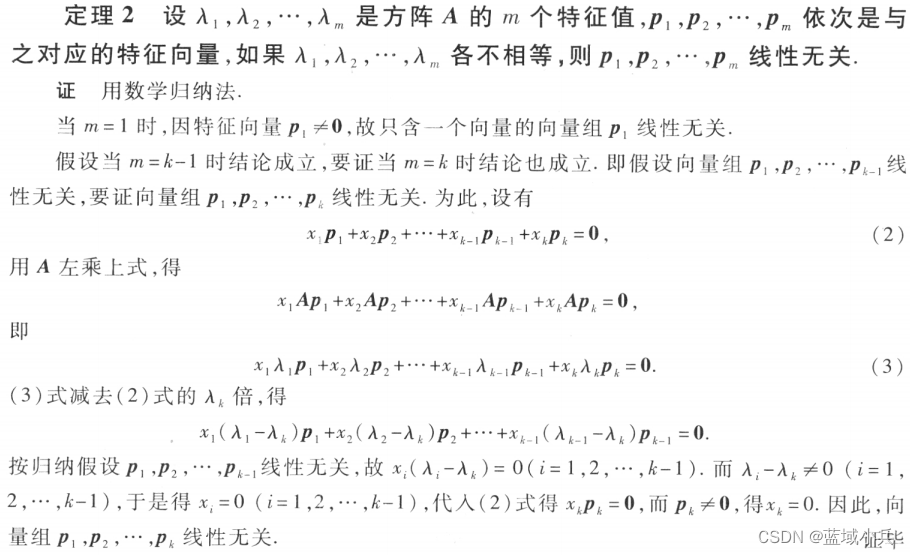• 对称矩阵正定的条件
千次阅读
2021-09-30 21:58:11

实对称矩阵合同于一个对角矩阵

任何n元二次型xTAx ，都可以通过坐标变换化为标准型。

n元二次型xTAx ，其中A是实对称矩阵，必存在正交变换x=Qy（Q是正交矩阵）使得xT A x 化为标准型。

A正定<==>所有特征值为正

> 设A是n阶正定阵，E是n阶单位阵，证明A+E的行列式大于1．

因为A是n阶正定阵，所以其特征值均大于0．
设λ为A的一个特征值，ξ为对应与λ的一个特征向量， 则：
（A+E）ξ=Aξ+ξ=λξ+ξ=（λ+1）ξ， 即λ+1为A+E的特征值．
注意到λ＞0， 故：A+E的特征值均大于1．
设A+E的特征值为：λ 1 ，λ 2 ，…，λ n ， 则 λ i ＞1，
从而：|A+E|=λ1 λ2 …λn ＞1．

设A为n阶实对称阵，且A3-3A2+5A-3E=O,证明A正定

证：设λ为A的任意一个特征向量ξ对应的特征值，则
(A³-3A²+5A-3E)ξ=(λ³-3λ²+5λ-3)ξ
=(λ³-λ²-2λ²+2λ+3λ-3)ξ
=(λ-1)(λ²-2λ+3)ξ=0
特征向量ξ≠0，故(λ-1)(λ²-2λ+3)=0，
得唯一实根λ=1(实对称矩阵的特征值都是实数)
即A的所有特征值都是正数，故实对称矩阵A正定。

设A,A-E都是n阶正定矩阵,证明E-A-1为正定矩阵

Aξ=aξ,a>0
(A-E)ξ=(a-1)ξ,a-1>0
(E-A-1)ξ=(1-1/a)ξ,有1-1/a>0
特征值均大于0，故正定

更多相关内容
• 将非正定对称矩阵转换为正定对称矩阵（即可逆矩阵）的函数。 一种特殊情况可能是协方差矩阵的求逆。 矩阵的特征分解用于向特征值 <= 0 添加一个小值。matlab
• 对输入的正定对称矩阵进行cholesky分解，只需要输入右三角矩阵部分即可
• 1，对称矩阵的特征值是实数。 证明如下：（我是用latex编辑的，这里不能显示公式，所以我只能用图片了。 上面的证明可以说明对称矩阵的特征值一定是实数！ 2、n阶方阵一定有n个特征跟（重跟按重数计算） 证明： 设A...

本文是为了在学习凸优化的时候遇到的一个问题展开讨论的。目的是能够明白凸优化的理论基础，或者尽可能的明白它的理论基础。

1，对称矩阵的特征值是实数。

证明如下：（我是用latex编辑的，这里不能显示公式，所以我只能用图片了。上面的证明可以说明对称矩阵的特征值一定是实数！

2、n阶方阵一定有n个特征跟（重跟按重数计算）

证明：

设A是一个n阶的方阵，它的特征多项式是一个关于符号lambda的一个n次多项式，根据代数基本定理，它可以唯一的分解成一次因式的乘积。所以一定有n个复数跟。

3、n阶实对称矩阵一定有n个实特征跟（重跟按重数计算）

由1和2便可以得到这个结论。

4、对称矩阵，从属于不同特征值的特征向量正交。

证明：5、设A为n阶对称矩阵，则必有正交矩阵P，使得P^{-1}AP = P’AP = B，其中B是以A的特征值为对角线元素的对角矩阵。

这个不证明。

6、n阶对称阵的k重特征值的特征空间的维数是k。

7、对称矩阵所有特征向量以及零向量可以组成的线性空间还是原空间！

8、总上结论，我们可以得到结论：

实对称矩阵是非负定矩阵的充分必要条件是它的所有特征值都非负！！！

实对称矩阵是正定矩阵的充分必要条件是它的所有特征值都大于0！！！


上面两个结论只需要用正定矩阵的定义和实对称矩阵的性质证明。

展开全文• 即当我们说一个矩阵是正定的，就已经默认它是一个对称矩阵。这一点似乎很多教材上存在不同的说法，或者并没有明确指出，但其实已经暗含了这个条件，因为在正定矩阵这一块，都是说有一个二次型。......

# 问题提出

今天做到一道线性代数的证明题，如下：

A , B A, B 都是 n n 阶正定矩阵，且 A B = B A AB=BA ，证明： A B AB 也是正定矩阵。

想了好半天没想出来，结果一翻答案，竟然是按照特征值证明的，两个矩阵相乘，特征值也相乘吗？ 一开始没想明白，顺着这个思路在网上找了半天，没找到答案，反倒是直接搜这个问题得到了一个能够理解的证明思路，下面记录一下证明过程，顺带这道题的一些扩展思路

# 理论准备

## 1 对称矩阵与反对称矩阵

首先需要明确的是，对称矩阵和反对称矩阵都是指方阵。另外，一般讨论的对称矩阵和反对称矩阵都是在定义在实数域的，即都是实矩阵

• 对称矩阵
A A n n 阶矩阵，且满足 A T = A A^T=A ，则 A A 为对称矩阵。因此，对于对称矩阵 A A ，应该满足 a i j = a j i ,       i , j = 1 , 2 , . . . , n . a_{ij}=a_{ji}, \, \, \space \space i,j=1,2,...,n.
• 反对称矩阵
A A n n 阶矩阵，且满足 A T = − A A^T=-A ，则 A A 为反对称矩阵。因此，对于反对称矩阵 A A ，应该满足 a i j = − a j i ,       i , j = 1 , 2 , . . . , n . a_{ij}=-a_{ji}, \, \, \space \space i,j=1,2,...,n. 反对称矩阵的对角元素都为0

根据对称矩阵和反对称矩阵的定义，可以得到一个重要的结论：

任意一个 n n 阶矩阵（方阵），都可以分解为一个 n n 阶对称矩阵 S S 和一个 n n 阶反对称矩阵 M M 之和

这个命题的证明思路非常简单：
A = A + A T 2 + A − A T 2 ,    令 S = A + A T 2 ， M = A − A T 2 A=\frac{A+A^T}{2}+\frac{A-A^T}{2},\space \space \space 令S=\frac{A+A^T}{2}，M=\frac{A-A^T}{2}
显然， S T = S , M T = − M S^T=S, M^T=-M ，故 M M 为对称矩阵， S S 为反对称矩阵，证毕。

其实对称是矩阵的一个非常重要的性质，但是从它的定义来看，似乎也没有什么能够得到的性质或推论，尤其是在矩阵乘法方面，即使是对称矩阵，乘了一个矩阵之后就不一定对称了，但是对于对称矩阵相乘，有一个可能会有用的结论。

A , B A, B 为对称矩阵，则 A B AB 为对称矩阵 ⇔ \Leftrightarrow A , B A, B 可交换（即 A B = B A AB=BA

证明思路如下：## 2 正定矩阵

首先需要明确的一点的是，正定矩阵是定义在实对称矩阵上的，即当我们说一个矩阵是正定的，就已经默认它是一个对称矩阵。这一点似乎很多教材上存在不同的说法，或者并没有明确指出，但其实已经暗含了这个条件，因为在正定矩阵这一块，都是说有一个二次型 A A …即已经将讨论范围限定在二次型了，即对称矩阵。

值得一提的是，为什么正定矩阵要定义在对称矩阵上？因为根据其特征值都大于0的判别方式，其实也有很多非对称矩阵满足这个条件。
在知乎上找到一个链接，虽然它底下的答案都是回答“正定矩阵是否都是对称矩阵” 这个问题，但个人觉得这些答案或许用来解释 “为什么正定矩阵都定义在对称矩阵上”。其中一个利用了上面证明的那个矩阵拆解的定理，其中值得注意的是，反对称矩阵的二次型为0（根据定义可以推得），因此，讨论一个 n n 阶矩阵的二次型问题，即使它对应的矩阵不是对称矩阵（即交叉项没有对半拆），最后都可以转化为对称矩阵的二次型问题，因此正定矩阵索性就定义在对称矩阵上。

如果加上对称这个条件，那么正定矩阵的判定与性质可以总结为以下几点：

• 常用的两种判别方式
• 特征值法：矩阵 A A 为对称矩阵 + A A 的所有特征值都大于0；
• 顺序主子式法：矩阵 A A 为对称矩阵 + A A 的各阶顺序主子式都大于0 ；
• 其他在证明题中常用的等价结论
• A A 为正定矩阵 ⇔ \Leftrightarrow 存在可逆矩阵 B B , 使得 A = B T B A=B^TB
• A A 为正定矩阵 ⇔ \Leftrightarrow A ⋍ E A\backsimeq E E E 为单位矩阵，有些教材用的是 I I
• A A 为正定矩阵 ⇔ \Leftrightarrow A A 的正惯性指数为 n n

# 问题证明

有了上述理论知识储备，我们再来看一下最开始提出的问题：

A , B A, B 都是 n n 阶正定矩阵，且 A B = B A AB=BA ，证明： A B AB 也是正定矩阵。

下面是证明过程：

证：
因为 A , B A, B n n 阶矩阵，则 A T = A , B T = B A^T=A, B^T=B ，又因为 A B = B A AB=BA ，故 A B AB 为对称矩阵，即满足正定矩阵的前提条件。
因 A , B A, B 为正定矩阵，故存在可逆矩阵 P P Q Q ，使得 A = P T P ,     B = Q T Q A=P^TP, \space \space \space B=Q^TQ .
由此得到：
Q A B Q − 1 = Q ( P T P ∗ Q T Q ) Q − 1 = Q ( P T P ) Q T = ( P Q T ) T ∗ ( P Q T ) QABQ^{-1}=Q(P^TP*Q^TQ)Q^{-1}=Q(P^TP)Q^T=(PQ^T)^T*(PQ^T)
显然， P Q T PQ^T 为可逆矩阵，故 ( P Q T ) T ∗ ( P Q T ) (PQ^T)^T*(PQ^T) 为正定矩阵，其特征值都大于0，而 A B AB 与矩阵 ( P Q T ) T ∗ ( P Q T ) (PQ^T)^T*(PQ^T) 相似，故其特征值相同，都大于0。故 A B AB 为正定矩阵，证毕。

展开全文矩阵乘法
• 通过分析对称正交矩阵对称正交对称正定矩阵的结构，利用矩阵的奇异值分解，导出了这种逆特征值问题的最小二乘解的表达式，以及这种逆特征值问题相容的充要条件和通解表达式。利用矩阵的极分解，导出了逆特征值...
• 从酉相似的角度证明实对称矩阵一定可以对角化，对角化之后对应的二次型一定大于0，因此实对称矩阵一定是正定矩阵。 第一张图说明了一个方阵A必定酉相似于一个上三角矩阵T，T的对角线元素就是A的特征值。且这里可以...

从酉相似的角度证明实对称矩阵一定可以对角化，对角化之后对应的二次型一定大于0，因此实对称矩阵一定是正定矩阵。
第一张图说明了一个方阵A必定酉相似于一个上三角矩阵T，T的对角线元素就是A的特征值。且这里可以无论特征值重复与否。第二张图证明了对于正规矩阵（就是AA=AA,*表示共轭转置）的矩阵来讲，A必定可以酉对角化。在第一张图中，如果限定实数矩阵范围，同样也成立，其实也就是矩阵化为行阶梯矩阵的高级表达。
在第二张图中，如果A是正规矩阵（实对称矩阵是正规矩阵的一种），则其相似的上三角矩阵也是正规矩阵，上三角矩阵是正规矩阵，则这个上三角矩阵就是一个对角矩阵（见铅字笔记）。

开始写了一大段发现自己不能给出实对称矩阵的代数重数为k的特征值对应有k个线性无关的特征向量的证明，不过写了好大一段不想删了，将就看吧。

## 一、实对称矩阵一定可以对角化

### 1.1 构造性证明与非构造性证明

数学证明方法可以分为构造性证明和非构造性证明，对于某个证明命题“存在 x x ，使得命题 F ( x ) F(x) 成立”，构造性证明是提出一种如何构造 x x 的方法，即遵循该方法，一定可以找到一个 x x 满足命题 F ( x ) F(x) ，而非构造性证明，则从逻辑上证明 x x 一定存在，至于如何得到 x x 则不再关心。

### 1.2 什么是对角化

对于实对称矩阵一定可以对角化这一命题，我们可以采用构造性证明的方法。
首先说明什么是对角化，对于矩阵 A A ，如果存在可逆矩阵，满足 P − 1 A P = Λ P^{-1}AP=\Lambda ，其中 Λ \Lambda 是对角矩阵，将 A A 变换得到对角矩阵 Λ \Lambda 这一过程就叫做对角化。满足 P − 1 A P = Λ P^{-1}AP=\Lambda 条件的 A A Λ \Lambda 矩阵其实就是满足 A A Λ \Lambda 相似，只不过现在与A相似的 Λ \Lambda 是对角矩阵。

### 1.3 如何对角化一个对称矩阵

从1.2可以知道，将 A A 对角化就是需要找到一个可逆矩阵 P P ，使得 P − 1 A P = Λ P^{-1}AP=\Lambda 。如果我们可以找到一种对所有对称矩阵通用的方法来找到可逆矩阵P，其实也就证明了所有实对称矩阵可以相似对角化。
我们只讨论其特征方程 ∣ A − λ E ∣ = 0 |A-λE|=0 的情况，因为在 ∣ A − λ E ∣ ≠ 0 |A-λE|\neq0 的情况下，不能进行对角化操作，见3.1
从3.1的推导中其实已经可以找到如何构造 P P ，就是将 P P 写成列向量分块矩阵。然后可以发现，求列向量 c o l i col_i 和对角矩阵对角元的过程就是求解矩阵A的特征向量和特征值的过程。也就是说，将 A A 的特征向量作为 P P 的列向量，其实就可以构造出 P P ，但是 P P 需要满足可逆这一条件，也就是说 P P 的列向量需要线性无关。

定理1 λ 1 , λ 2 , . . . , λ m \lambda_1,\lambda_2,...,\lambda_m 是方阵A的 m m 个特征值， p 1 , p 2 , . . . , p m p_1,p_2,...,p_m 依次是与之对应的特征向量，如果 λ 1 , λ 2 , . . . , λ m \lambda_1,\lambda_2,...,\lambda_m 各不相等，则 p 1 , p 2 , . . . , p m p_1,p_2,...,p_m 线性无关，证明见3.2

定理二 λ k \lambda_k 是对称矩阵 A A k k 重特征值，那么一定可以找到 k k 个对应于特征值 λ k \lambda_k 的线性无关的特征向量证明见3.3

从定理一和定理二可以知道，对于对称矩阵，我们可以找出 n n 个线性无关的特征向量来构造 P P 。因为对应于不同特征值的特征向量线性无关，对应于同一特征值的特征向量可以得到对应重数个线性无关向量。因此总共有 n n 个线性无关的特征向量用于构造矩阵 P P 。最后将 A A 进行对角化。

## 二、实对称矩阵一定是正定矩阵

从前面我们可以知道实对称矩阵可以通过构造可逆矩阵P进行对角化，但是实对称矩阵

## 三、后注

### 3.1 特征方程只有零解的情况无法对角化

∣ A − λ E ∣ = 0 |A-λE|=0 ，也即(A-λE)x=0这一齐次方程只有零解（翻一翻线性代数书中齐次线性方程组零解非零解的条件，题外话：也就意味着矩阵A对于任何向量x进行变换都会改变其方向，从这个意义上来说，这个矩阵就不存在特征向量和特征值，因为特征向量的几何意义就是经过这个矩阵变换不改变方向的向量，零向量不存在方向，因此对于此类矩阵讲特征值和特征向量是没有意义的）。将前面的相似对角化矩阵 P − 1 A P = Λ P^{-1}AP=\Lambda 可以变换成 A P = P Λ AP=P\Lambda ，将P写成列向量组合成的分块矩阵形式，\Lambda写成元素组合形式得到：
A [ c o l 1 , c o l 2 , . . . , c o l n ] = [ c o l 1 , c o l 2 , . . . , c o l n ] ( λ 1 λ 2 ⋱ λ n ) A[col_{1}, col_2,...,col_n]=[col_1,col_2,...,col_n]\left(\begin{array}{cccc} \lambda_{1} & & & \\ & \lambda_{2} & & \\ & & \ddots & \\ & & & \\ & & & \lambda_{n} \end{array}\right)
 [ A c o l 1 , A c o l 2 , . . . , A c o l n ] = [ λ 1 c o l 1 , λ 2 c o l 2 , . . . , λ n c o l n ] [Acol_1,Acol_2,...,Acol_n]=[\lambda_1col_1, \lambda_2col_2,...,\lambda_ncol_n]
即有 A c o l i = λ i c o l i Acol_i=\lambda_icol_i ，由于 A A 不存在特征值和特征向量，对于 A c o l i = λ i c o l i Acol_i=\lambda_icol_i 只有零解，因此 c o l i = 0 col_i=\bold{0} 零向量。因此P为零矩阵。而零矩阵不存在逆矩阵，与前面的逆矩阵使用矛盾，因此当矩阵A的特征方程只有零解时，不能进行对角化。

### 3.2 定理一的证明

定理1 λ 1 , λ 2 , . . . , λ m \lambda_1,\lambda_2,...,\lambda_m 是方阵A的 m m 个特征值， p 1 , p 2 , . . . , p m p_1,p_2,...,p_m 依次是与之对应的特征向量，如果 λ 1 , λ 2 , . . . , λ m \lambda_1,\lambda_2,...,\lambda_m 各不相等，则 p 1 , p 2 , . . . , p m p_1,p_2,...,p_m 线性无关，证明见3.2
证明出处见同济大学出版的工程数学线性代数第六版P123### 3.3 定理二的证明

定理二 λ k \lambda_k 是对称矩阵 A A k k 重特征值，那么对应于 λ k \lambda_k 的特征向量构成的向量组的秩为 k k 证明见3.3

展开全文• 做最小二乘法常常会遇到正定对称矩阵求逆的问题。 本程序只包含两个参数， 1、double *B //输入，正定对称矩阵的首地址；输出，存放逆矩阵 2、矩阵的阶数
• 本文主要针对线性代数中的正定矩阵、实对称矩阵、矩阵特征值分解以及矩阵 SVD 分解进行总结。 如果你对这篇文章可感兴趣，可以点击「【访客必读 - 指引页】一文囊括主页内所有高质量博客」，查看完整博客分类与对应...矩阵SVD分解 矩阵正交对角化
• 1,对称矩阵正定矩阵 对于实对称矩阵,其特征值都是实数,其不同特征值对应的特征向量相互正交. 所以对于是对称矩阵,其特征矩阵可以用正交矩阵代替,也就是常说的对称矩阵可以用正交矩阵进行相似对角化, 并且Q的逆...机器学习
• 正定矩阵作为一类特殊的对称矩阵，它又有哪些特性？本文的相关知识：正交向量和正交矩阵 (格拉姆-施密特正交化)01 对称矩阵对称矩阵是最重要的矩阵之一，对于对称矩阵来说， 。矩阵的特殊性也表现在特征值和特征...
• 方法 1：尝试 Cholesky 分解检查矩阵是否为对称正定矩阵的最...此方法不要求矩阵为对称矩阵也能成功进行测试(如果矩阵不对称，则分解将会失败)。A = [1 -1 0; -1 5 0; 0 0 7]A = 3×31 -1 0-1 5 00 0 7try chol(A)di...
• 线性代数之 实对称矩阵，正交对角化，二次型与正定矩阵前言实对称矩阵正交对角化二次型正定矩阵实对称矩阵正定判断条件后记 前言 终于快到矩阵分解了。在矩阵分解前，最后一个内容是实对称矩阵，二次型和正定矩阵...机器学习
• 对称正定矩阵的三角分解 设 A\ A A为n阶对称正定矩阵，则 A\ A A可以分解为一个单位下三角矩阵 L~\ \tilde{L} L~和一个上三角矩阵 U~\ \tilde{U} U~的乘积：  A=L~U~\...matlab
• 研究了下列问题:给定X∈Rn×p,B∈Rp×p,A0∈SRn>×0n( p
• 一个nXn实矩阵A称为半正定,如果对每个n维非零实向量x,均有xAx>0本文给出了两个半正定,未必对称矩阵为半正定的充要条件
• 还有一门学科，叫“矩阵论”，在这个框架下，认为正定矩阵不一定是对称矩阵。有一门学科，叫“线性代数”，在这个框架下，认为正定矩阵一定是对称矩阵。就要求它必须是：埃尔米特矩阵，在实数上就是对称阵的意思。机器学习 数据挖掘 统计学习
• 在很多问题（如非线性 LS）中，我们需要确保矩阵是正定的。 此函数返回一个正定对称矩阵matlab
• 设二次型，是对称矩阵，如果对任何，都有（显然），则称为正定二次型，并称对称矩阵正定的。 2 MATLAB随机生成正定矩阵 1）用随机函数生成对称正定矩阵 rand(m,n)：随机生成每个元素在0~1之间的m行n列的...
• ## 对称矩阵及正定性

千次阅读 2019-05-21 06:56:09
对称阵是非常重要的矩阵，对于实对称矩阵，其特征值也为实数，且特征向量是垂直的。注意这里的垂直是指：如果特征值互不相同，那么每个特征值对应的特征向量是在一条线上，那些线之间总是垂直的；如果特征值重复，那...
• 两种生成对称正定矩阵的方法 1. pascal() P = pascal(n) 返回 n 阶帕斯卡矩阵。P 是一个对称正定矩阵，其整数项来自帕斯卡三角形。 帕斯卡矩阵有非常好的性质。首先各个矩阵中元素都是从1开始比较小的正整数，便于...
• Sigma为一个对称矩阵，但非半正定，进行choleskey分解后报error > chol(Sigma) Error in chol.default(Sigma) : the leading minor of order 14 is not positive definite 结合tryCatch判断矩阵半正定 try...r语言
• M = input('请输入一个矩阵用来判断：') if M==M' disp('对称矩阵:是') else; disp('对称矩阵:不是') ... disp('正定对称矩阵：是') else; disp('正定对称矩阵：不是') end #运行结果 ...
• 本文考虑以下问题:问题Ⅰ:给定G∈Rn×p,X,B∈Rn×m,求A∈GSRn≥×0n使得AX = B,其中:GSRn≥×0n = {A∈Rn×n| xTAx≥0且xT( A- AT) =0,∨x∈...讨论了问题Ⅰ与问题Ⅱ有解的充要条件,并在有解时给出了通解的一般表达式。...